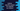# How to read the content of a specific column of csv file in Python## Python read the content of a specific csv file column :

Python provides csv module to do read-write operations on a csv file. We can use this module to read the contents line by line or with a slight change, we can read the contents of a specific column.

Let’s consider the below csv file :

``````Name, Age, Marks
Alex, 11, 20
Bob, 12, 21
Charlie, 11, 23``````

Now, to read the rows, we can do something like below :

``````import csv
with open('sample.csv', 'r') as file:
for line in csv_reader:
print(line)``````

Plain and simple ! The content is in a sample.csv file and we are reading the file line by line. It will print the below output :

``````['Name', ' Age', ' Marks']
['Alex', ' 11', ' 20']
['Bob', ' 12', ' 21']
['Charlie', ' 11', ' 23']``````

Each line is a list of strings. If we want to print the value for a specific column, we can use index to access that. For example, the below program shows the Age of each student :

``````import csv
with open('sample.csv', 'r') as file:
for line in csv_reader:
print(line)``````

Output :

`````` Age
11
12
11``````

### Using pandas :

Another way to do the same thing is by using pandas. Before that, go to this link and install Anaconda if you don’t have it on your machine or you can use pip to install it directly.

You can use pandas like any other module. Below is the method used to read column data from a csv file :

``read_csv(file, usecols=column_list)``

where, file is the csv file to read data and column_list is the list of all column names.

Let’s take a look at the below example :

``````import pandas

pandas_data = pandas.read_csv('sample.csv',usecols = ["Age"])

print(pandas_data)``````

sample.csv contains the below data :

``````Name,Age,Marks
Alex, 11, 20
Bob, 12, 21
Charlie, 11, 23``````

It prints the below output :

``````0   11
1   12
2   11``````

Make sure not to add any blank space in the title, e.g. if the title is Name, Age, Marks, you need to add one space to the usecols list element.